# MP Board Class 8th Maths Solutions Chapter 11 Mensuration Ex 11.4

## MP Board Class 8th Maths Solutions Chapter 11 Mensuration Ex 11.4

Question 1.
Given a cylindrical tank, in which situation will you find surface area and in which situation volume.
(a) To find how much it can hold.
(b) Number of cement bags required to plaster it.
(c) To find the number of smaller tanks that can be filled with water from it.
Solution:
(a) To find how much a cylinder can hold, we need to find the volume of the cylindrical tank.
(b) To find the number of cement bags required to plaster the tank, we need to find the surface area of the cylindrical tank.
(c) To find the number of smaller tanks that can be filled with water from the bigger tank, we need to find the volume of the big cylindrical tank and one small tank.Question 2.
Diameter of cylinder A is 7 cm, and the height is 14 cm. Diameter of cylinder B is 14 cm and height is 7 cm. Without doing any calculations can you suggest whose volume is greater ? Verify it by finding the volume of both the cylinder. Check whether the cylinder with greater volume also has greater surface area?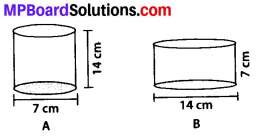Solution:
∵ Volume of cylinder = πr2h
Now, radius of cylinder B is double the radius of cylinder A and height of cylinder B is half the height of A. Due to the square of radius, the radius will assert greater impact in the volume of cylinder than the height. So, volume of cylinder B will be greater.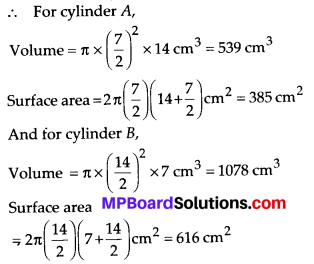Hence, cylinder B has both, greater volume and greater surface area.Question 3.
Find the height of a cuboid whose base area is 180 cm2 and volume is 900 cm3 ?
Solution:
We have, base area = 180 cm2,
Volume = 900 cm3
To find, height of a cuboid = h cm, say
We know, Volume = Base area × Height of a cuboid
⇒ 900 = 180 × h ⇒ h = 5 cm.

Question 4.
A cuboid is of dimensions 60 cm × 54 cm × 30 cm. How many small cubes with side 6 cm can be placed in the given cuboid?
Solution:
We have,
Big cuboid dimensions = 60 cm × 54 cm × 30 cm
Side of a small cube = 6 cm
Number of cubes that can be placed in the given cuboid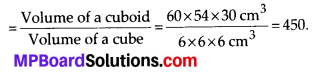Question 5.
Find the height of the cylinder whose volume is 1.54 m3 and diameter of the base is 140 cm?
Solution:
We have, volume of the cylinder = 1.54 m3 = 1.54 × 106 cm3
Diameter = 140 cm,
Radius = 140 ÷ 2 = 70 cm
∵ volume of the cylinder = πr2h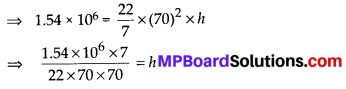⇒ 100 cm = h
∴ Height of the cylinder is 100 cm.Question 6.
A milk tank is in the form of cylinder whose radius is 1.5 m and length is 7 m. Find the quantity of milk in litres that can be stored in the tank?
Solution:
We have, radius of the cylindrical tank = 1.5 m and length = 7 m
∴ Volume of tank = πr2h = π(1 .5)2 × 7
= 49.5 m3 = 49500 litres. [∵ 1 m3 = 1000 L]

Question 7.
If each edge of a cube is doubled,
(i) how many times will its surface area increase?
(ii) how many times will its volume increase?
Solution:
Let the edge of the cube be a cm.
After doubling the length, the edge becomes 2a cm.
Surface area of old cube = 6a2 and volume of old cube = a3
Surface area of new cube = 6(2a)2 = 24 a2 and volume of new cube = (2a)3 = 8a3
Hence, surface area increases 4 times and volume increases 8 times if the edge of a cube is doubled.Question 8.
Water is pouring into a cuboidal reservoir at the rate of 60 litres per minute. If the volume of reservoir is 108 m3, find the number of hours it will take to fill the reservoir.
Solution:
We have,
volume of reservoir = 108 m3 = 108 × 103 L
[∵ 1 m3 = 103 L]
Rate of pouring water = 60 L/minute
Time to fill the reservoir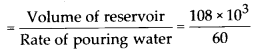= 1.8 × 103 min. = 1800 min.= 30 hrs.